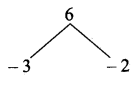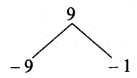## Tamilnadu Samacheer Kalvi 10th Maths Solutions Chapter 3 Algebra Ex 3.13

10th Maths Exercise 3.13 Samacheer Kalvi Question 1.
Determine the nature of the roots for the following quadratic equations
(i) 15.x2 + 11.x + 2 = 0
(ii) x2 – x – 1 = 0
(iii) $$\sqrt{2} t^{2}$$ – 3t + $$3 \sqrt{2}$$ = 0
(iv) 9y2 – $$6 \sqrt{2} y$$ + 2 = 0
(v) 9a2b2x2 – 24abcdx + 16c2d2 = 0 a ≠ 0, b ≠ 0
Solution:
(i) 15x2 + 11x + 2 = 0 comparing with ax2 + bx + c = 0.
Here a = 15, 6 = 11, c = 2.
Δ = b2 – 4ac
= 112 -4 × 15 × 2
= 121 – 120
= 1 > 1.
∴ The roots are real and unequal.

(ii) x2 – x – 1 = 0,
Here a = 1, b = -1, c = -1 .
Δ = b2 – 4ac
= (-1)2 – 4 × 1 × -1
= 1 + 4 = 5 > 0.
∴ The roots are real and unequal.

(iii) $$\sqrt{2} t^{2}$$ – 3t + $$3 \sqrt{2}$$ = 0
Here a = $$\sqrt{2}$$, b = -3, c = $$3\sqrt{2}$$
Δ = b2 – 4ac
= (-3)2 – 4 × $$\sqrt{2}$$ × $$3\sqrt{2}$$
= 9 – 24 = -15 < 0.
∴ The roots are not real.

(iv) 9y2 – $$6 \sqrt{2} y$$ + 2 = 0
a = 9, b = $$6\sqrt{2}$$ , c = 2
Δ = b2 – 4ac
= ($$6\sqrt{2}$$)2 – 4 × 9 × 2
= 36 × 2 – 72
= 72 – 72 = 0
∴ The roots are real and equal.

(v) 9a2b2x2 – 24abcdx + 16c2d2 = 0
Δ = b2 – 4ac
= (-24abcd)2 – 4 × 9a2b2 × 16c2d2
= 576a2b2c2d2 – 576a2b2c2d2
= 0
∴ The roots are real and equal.

Ex 3.13 Class 10 Samacheer Question 2.
Find the value(s) of ‘A’ for which the roots of the following equations are real and equal.
(i) (5k – 6)x2 + 2kx + 1 = 0
Here a = 5k – 6 ; b = 2k and c = 1
Since the equation has real and equal roots ∆ = 0.∴ b2 – 4ac = 0
(2k)2 – 4(5k – 6) (1) = 0
4k2 – 20k + 24 = 0
(÷ 4) ⇒ k2 – 5k + 6 = 0
(k – 3) (k – 2) = 0
k -3 = 0 or k – 2 = 0
k = 3 or k = 2
The value of k = 3 or 2

(ii) kx2 + (6k + 2)x + 16 = 0
Here a = k, b = 6k + 2; c = 16
Since the equation has real and equal roots∆ = 0
b2 – 4ac = 0
(6k + 2)2 – 4(k) (16) = 0
36k2 + 4 + 24k – 4(k) (16) = 0
36k2 – 40k + 4 = 0
(÷ by 4) ⇒ 9k2 – 10k + 1 = 0
9k2 – 9k – k + 1 = 0
9k(k – 1) – 1(k – 1) = 0
9k (k – 1) -1 (k – 1) = 0
(k – 1) (9k – 1) = 0
k – 1 or 9k – 1 = 0
k = 1 or k = $$\frac { 1 }{ 9 }$$
The value of k = 1 or $$\frac { 1 }{ 9 }$$

10th Maths Exercise 3.13 Solution Question 3.
If the roots of (a – b)x2 + (b – c)x + (c – a) = 0 are real and equal, then prove that b, a, c are in arithmetic progression.
Solution:
(a – b)x2 + (b – c)x + (c – a) = 0
A = (a – b), B = (b – c), C = (c – a)
Δ = b2 – 4ac = 0
⇒ (b – c)2 – 4(a – b)(c – a)
⇒ b2 – 2bc + c2 -4 (ac – bc – a2 + ab)
⇒ b2 – 2bc + c2 – 4ac + 4bc + 4a2 – 4ab = 0
⇒ 4a2 + b2 + c2 + 2bc – 4ac – 4ab = 0
⇒- (-2a + b + c)2 = 0 [∵ (a + b + c) = a2 + b2 + c2 + 2ab + 2bc + 2ca)]
⇒ 2a + b + c = 0
⇒ 2 a = b + c
∴ a, b, c are in A.P.

10th Maths Exercise 3.13 Question 4.
If a, b are real then show that the roots of the equation
(a – b)x2 – 6(a + b)x – 9(a – b) = 0 are real and unequal.
(a – b)x2 – 6(a + b)x – 9(a – b) = 0
Here a = a – b ; b = – 6 (a + b); c = – 9 (a – b)
∆ = b2 – 4ac
= [- 6(a + b)]2 – 4(a – b)[-9(a – b)]
= 36(a + b)2 + 36(a – b)(a – b)
= 36 (a + b)2 + 36 (a – b)2
= 36 [(a + b)2 + (a – b)2]
The value is always greater than 0
∆ = 36 [(a + b)2 + (a – b)2] > 0
∴ The roots are real and unequal.

Exercise 3.13 Question 5.
If the roots of the equation (c2 – ab)x2 – 2(a2 – bc)x + b2 – ac = 0 are real and equal prove that either a = 0 (or) a3 + b3 + c3 = 3abc.
Solution:
(c2 – ab)x2 – 2(a2 – bc)x + (b2 – ac) – 0
Δ = B2 – 4AC = 0 (since the roots are real and equal)
⇒ 4(a2′ – bc)2 – 4 (c2 – ab)(b2 – ac) = 0
⇒ 4(a4 – 2a2bc + b2c2) – 4(c2b2 – ab3 – ac3 + a2bc) = 0
⇒ 4a4 + 4b2c2 – 8a2bc – 4c2b2 + 4ab3 + 4ac3 – 4a2bc = 0
⇒ 4a4+ 4ab3 + 4ac3 – 4a2bc – 8a2bc = 0
⇒ 4a [a3 + b3 + c3] = 0 or a = 0
⇒ a = 0 or [a3 + b3 + c3 – 3abc] = 0
⇒ a3 + b3 + c3 – 3abc = 0
⇒ a3 + b3 + c3 = 3abc or a = 0
Hence proved.